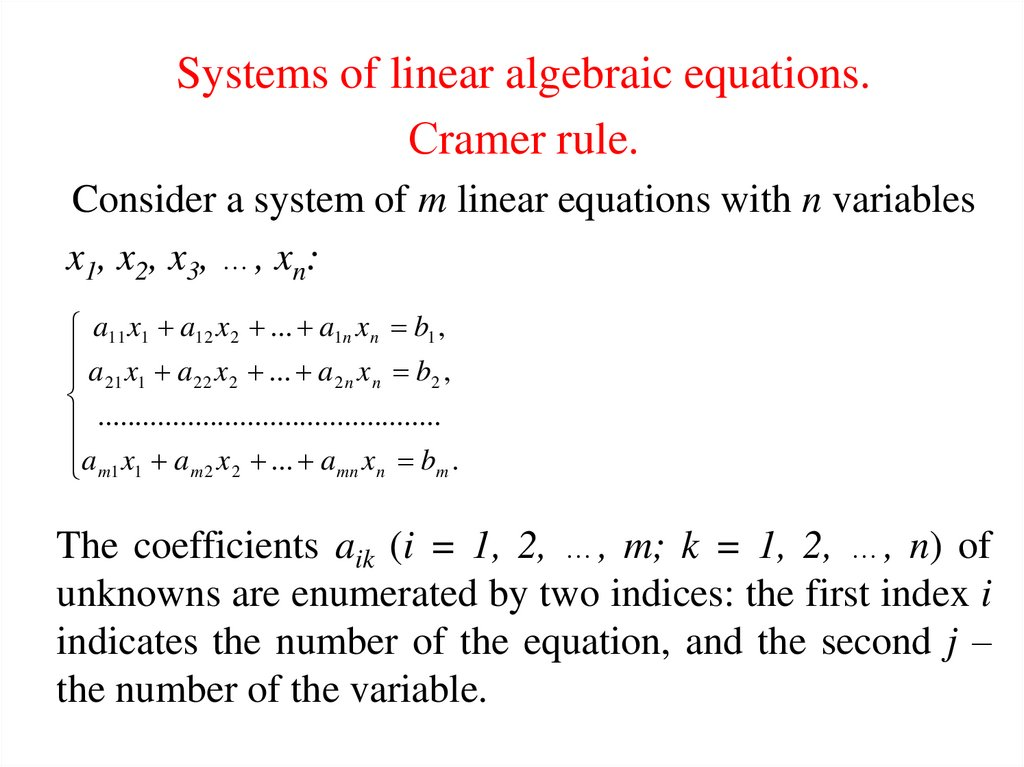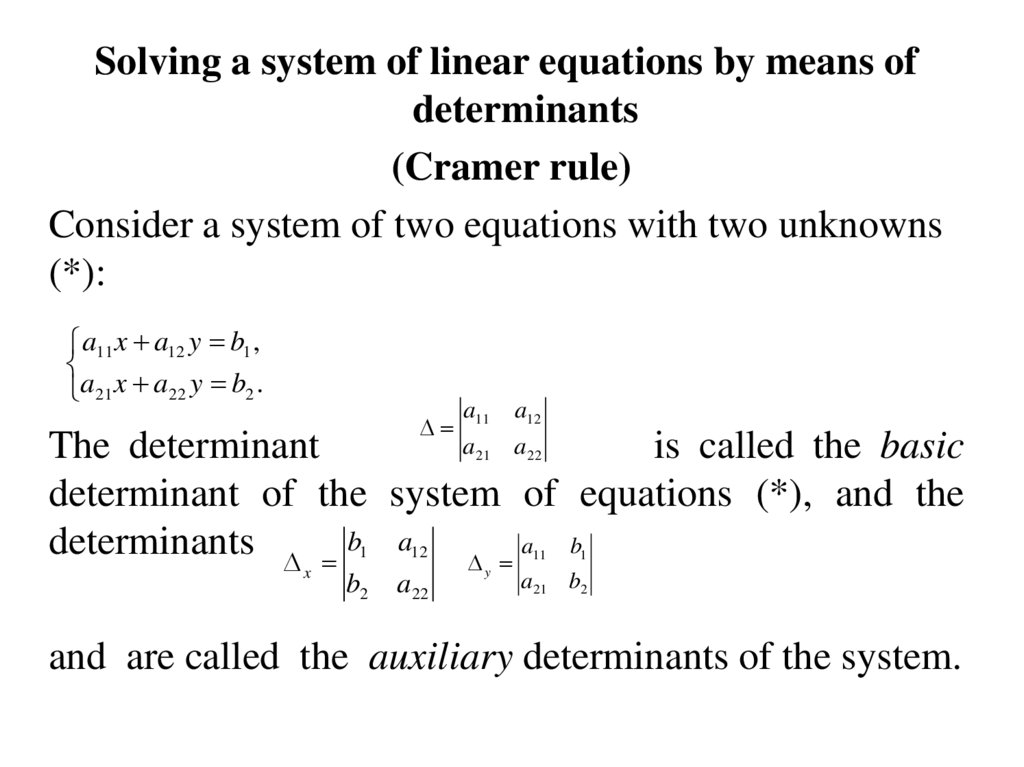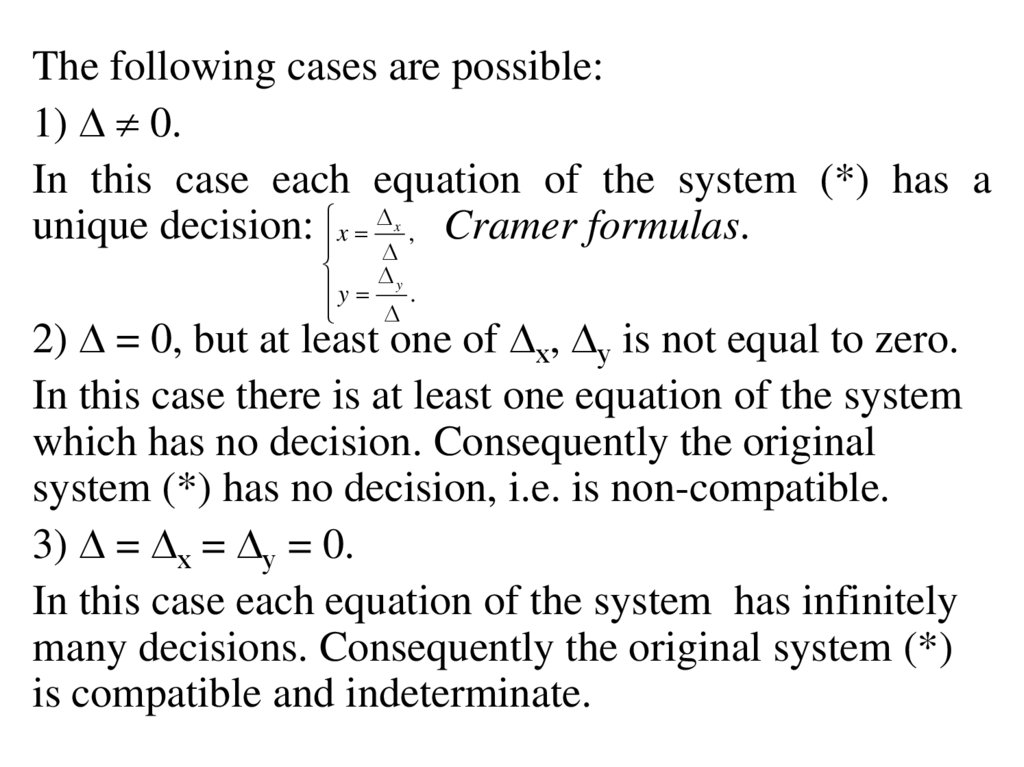# Systems of linear algebraic equations

## 1.

Systems of linear algebraic equations.
Сramer rule.
Consider a system of m linear equations with n variables
x1, x2, x3, …, xn:
a11 x1 a12 x 2 ... a1n x n b1 ,
a x a x ... a x b ,
21 1
22 2
2n n
2
..............................................
a m1 x1 a m 2 x 2 ... a mn x n bm .
The coefficients aik (i = 1, 2, …, m; k = 1, 2, …, n) of
unknowns are enumerated by two indices: the first index i
indicates the number of the equation, and the second j –
the number of the variable.

## 2.

Solving a system of linear equations by means of
determinants
(Cramer rule)
Consider a system of two equations with two unknowns
(*):
a11 x a12 y b1 ,
a21 x a22 y b2 .
a11
a12
a
a
The determinant
is called the basic
determinant of the system of equations (*), and the
determinants b1 a12 a b
21
22
11
x
b2
a22
y
1
a21 b2
and are called the auxiliary determinants of the system.

## 3.

The following cases are possible:
1) 0.
In this case each equation of the system (*) has a
unique decision: x , Cramer formulas.
x
y y .
2) = 0, but at least one of х, у is not equal to zero.
In this case there is at least one equation of the system
which has no decision. Consequently the original
system (*) has no decision, i.e. is non-compatible.
3) = х = у = 0.
In this case each equation of the system has infinitely
many decisions. Consequently the original system (*)
is compatible and indeterminate.

## 4.

Example 1. Solve the system
2 x 3 y 7,
3x 4 y 2.
Solution: 1) Compute the basic determinant of the system
2 3
3
4
8 9 17.
2) Compute the auxiliary determinants of the system:
x
7 3
2
28 6 34; y
2 7
4 21 17.
3 2
= 17 0 x x 34 2; y y 17 1.
17
17
4
2 ( 2) 3 1 7
3 ( 2) 4 1 2

## 5.

a11 x a12 y a13 z b1
a 21 x a 22 y a 23 z b2
a x a y a z b
32
33
3
31
the Cramer formulas are deduced analogously and have the
following form:
y
x
x
, y
,
z
z
a11
a12
a13
b1
a12
a13
a 21
a 22
a 23 0, x b2
a 22
a31
a32
a33
a32
b3
a11
a11
a12
b1
a 23 , y a 21 b2
a 23 , z a 21
a 22
b2 .
a33
a33
a32
b3
a31
b1
b3
a13
a31

## 6.

Example 2. Solve the system of equations:
2 x 3 y z 5,
x 2 y 3 z 4,
3 x y 2 z 1.
Solution. Compute the basic and auxiliary determinants of the
system:
2
3
1
3 8 27 1 6 6 6 18 0 x 4
2
3
1
2
5 3
1
1
2
5
2
1
1
3 20 9 4 2 24 1 18,
2
1
y 1 4 3 16 45 1 12 10 6 36,
3
2
z 1
3
1
2
3 5
2
1
4 4 36 5 30 3 8 54.
1
x
y 36
x 18
54
1; y
2; z z
3.
18
18
18